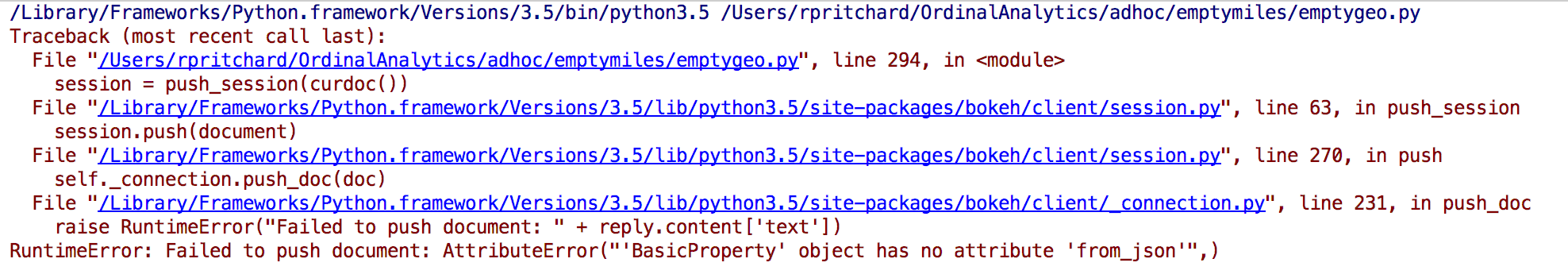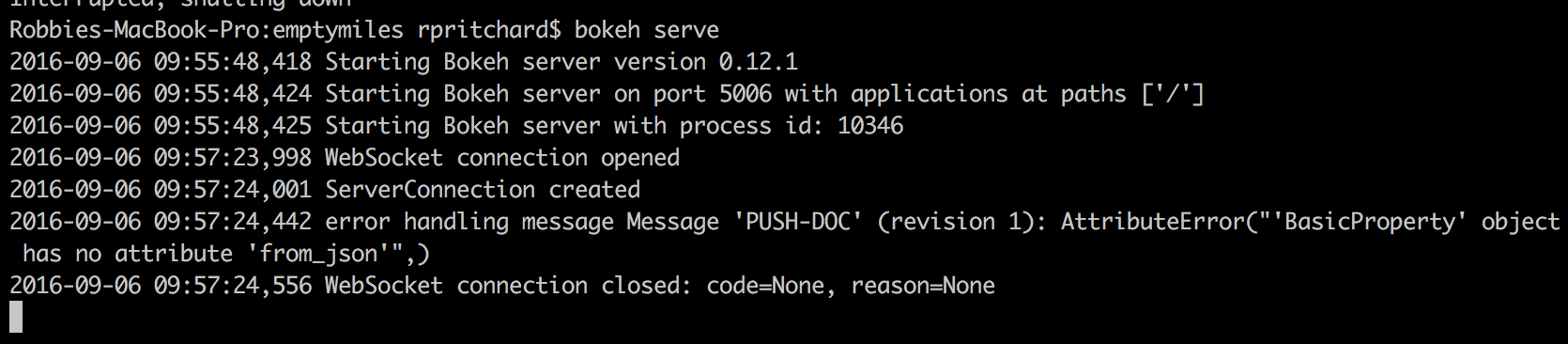# GMap Plot Bokeh Errors

I was getting no response on my last post.

Bokeh version 0.12.1

OSX 10.11.6

I am getting an AttributeError("‘BasicProperty’ object has no attribute ‘from_json’",) and I don’t know where its coming from. Attached are the log files from terminal and pycharm.

Here is some example code that gives the same error.

from bokeh.plotting import Figure, ColumnDataSource, show, vplot

from bokeh.io import output_file

from bokeh.models import (Slider, CustomJS, GMapPlot,

``````                       GMapOptions, DataRange1d, Circle, Line)
``````

import numpy as np

from bokeh.plotting import curdoc, figure

from bokeh.layouts import row, column, widgetbox

from bokeh.client import push_session

output_file(“path.html”)

r = 0.000192

x1 = np.linspace(-1,1,100)*r

x2 = np.linspace(1,-1,100)*r

x = np.hstack((x1,x2))

f = lambda x : np.sqrt(r2 - x2)

y1 = f(x1)

y2 = -f(x2)

y = np.hstack((y1,y2))

init_x = 40.233688

init_y = -111.646784

lon = init_x + x

lat = init_y + y

# Initialize data sources.

location = ColumnDataSource(data=dict(x=[lon], y=[lat]))

path = ColumnDataSource(data=dict(x=lon, y=lat))

# Initialize figure, path, and point

“”"I haven’t been able to verify that the GMapPlot code below works, but

this should be the right thing to do. The zoom may be totally wrong,

but my latlng points should be a path around a roundabout.

“”"

options = GMapOptions(lat=40.233681, lng=-111.646595, map_type=“roadmap”, zoom=15)

fig = GMapPlot(x_range=DataRange1d(), y_range=DataRange1d(), map_options=options,api_key=‘XXXXXXXXXXXXXXXX’)

# fig = Figure(plot_height=600, plot_width=600)

c = Circle(x=‘x’, y=‘y’, size=10)

p = Line(x=‘x’, y=‘y’)

# Slider callback

callback = CustomJS(args=dict(location=location, path=path), code="""

`````` var loc = location.get('data');

var p = path.get('data');

t = cb_obj.get('value');

/* set the point location to the path location that

corresponds to the slider position */

loc['x'] = p['x'][t];

loc['y'] = p['y'][t];

location.trigger('change');
``````

“”")

# ‘end’ has to be the length of the array of path points.

slider = Slider(start=0, end=200, step=1, callback=callback)

layout = column(fig, slider)

curdoc().clear()

session = push_session(curdoc())

session.show()

session.loop_until_closed()

``

**Sarah Birds - Response **

I’m not sure what’s going on, but I’ve started a little debugging

If you run bokeh-serve with --log-level=debug

You see the source of the error is:

File “/Users/caged/Dev/bokeh/bokeh/bokeh/core/properties.py”, line 1236, in from_json

``````attrs[name] = prop.from_json(value, models)
``````

AttributeError: ‘BasicProperty’ object has no attribute ‘from_json’

I stuck a print statement in to print out “prop” and it always prints out a GMapOptions value - although not always the same one - which makes sense because GMapOptions a dictionary.

e.g.

zoom:Int

lng:Float

lat:Float

I think there’s some kind of serialization problem with GMapPlot and the server (although maybe it’s wider). Hopefully Bryan has some thoughts.Based on Sarah's observations, I'd agree this seems like a bug that is particular to the serialization of MapOptions. In which case the next step is to file a bug report on GitHub. Fixing it will probably take some time, however, as there are many priorities and few people to work on them. As an immediate workaround I would suggest looking at using a regular Plot with a TileProvider layer:

There are a few tile providers built-in already:

CartoDB is probably a good choice.

Thanks,

Bryan

···

On Sep 8, 2016, at 7:49 AM, Robbie Pritchard <[email protected]> wrote:

I was getting no response on my last post.

Bokeh version 0.12.1

OSX 10.11.6

I am getting an AttributeError("'BasicProperty' object has no attribute 'from_json'",) and I don't know where its coming from. Attached are the log files from terminal and pycharm.

Here is some example code that gives the same error.

from bokeh.plotting import Figure, ColumnDataSource, show, vplot

from bokeh.io import output_file

from bokeh.models import (Slider, CustomJS, GMapPlot,

GMapOptions, DataRange1d, Circle, Line)

import numpy as np

from bokeh.plotting import curdoc, figure

from bokeh.layouts import row, column, widgetbox

from bokeh.client import push_session

output_file("path.html")

r = 0.000192

x1 = np.linspace(-1,1,100)*r

x2 = np.linspace(1,-1,100)*r

x = np.hstack((x1,x2))

f = lambda x : np.sqrt(r**2 - x**2)

y1 = f(x1)

y2 = -f(x2)

y = np.hstack((y1,y2))

init_x = 40.233688

init_y = -111.646784

lon = init_x + x

lat = init_y + y

# Initialize data sources.

location = ColumnDataSource(data=dict(x=[lon], y=[lat]))

path = ColumnDataSource(data=dict(x=lon, y=lat))

# Initialize figure, path, and point

"""I haven't been able to verify that the GMapPlot code below works, but

this should be the right thing to do. The zoom may be totally wrong,

but my latlng points should be a path around a roundabout.

"""

options = GMapOptions(lat=40.233681, lng=-111.646595, map_type="roadmap", zoom=15)

fig = GMapPlot(x_range=DataRange1d(), y_range=DataRange1d(), map_options=options,api_key='XXXXXXXXXXXXXXXX')

# fig = Figure(plot_height=600, plot_width=600)

c = Circle(x='x', y='y', size=10)

p = Line(x='x', y='y')

# Slider callback

callback = CustomJS(args=dict(location=location, path=path), code="""

var loc = location.get('data');

var p = path.get('data');

t = cb_obj.get('value');

/* set the point location to the path location that

corresponds to the slider position */

loc['x'] = p['x'][t];

loc['y'] = p['y'][t];

location.trigger('change');

""")

# The way I have written this, 'start' has to be 0 and

# 'end' has to be the length of the array of path points.

slider = Slider(start=0, end=200, step=1, callback=callback)

layout = column(fig, slider)

curdoc().clear()

session = push_session(curdoc())

session.show()

session.loop_until_closed()

Sarah Birds - Response

I'm not sure what's going on, but I've started a little debugging

If you run bokeh-serve with --log-level=debug

You see the source of the error is:

File "/Users/caged/Dev/bokeh/bokeh/bokeh/core/properties.py", line 1236, in from_json

attrs[name] = prop.from_json(value, models)

AttributeError: 'BasicProperty' object has no attribute 'from_json'

I stuck a print statement in to print out "prop" and it always prints out a GMapOptions value - although not always the same one - which makes sense because GMapOptions a dictionary.

e.g.

zoom:Int

lng:Float

lat:Float

I think there's some kind of serialization problem with GMapPlot and the server (although maybe it's wider). Hopefully Bryan has some thoughts.

--
You received this message because you are subscribed to the Google Groups "Bokeh Discussion - Public" group.
To unsubscribe from this group and stop receiving emails from it, send an email to [email protected].
To post to this group, send email to [email protected].
To view this discussion on the web visit https://groups.google.com/a/continuum.io/d/msgid/bokeh/878b0af5-653f-4f0f-b797-12bf85289197%40continuum.io.
<Screen Shot 2016-09-06 at 9.57.33 AM.png><Screen Shot 2016-09-06 at 9.59.17 AM.png>

Thanks Bryan, I filed the bug.

···

On Thursday, September 8, 2016 at 8:12:19 AM UTC-5, Bryan Van de ven wrote:

Based on Sarah’s observations, I’d agree this seems like a bug that is particular to the serialization of MapOptions. In which case the next step is to file a bug report on GitHub. Fixing it will probably take some time, however, as there are many priorities and few people to work on them. As an immediate workaround I would suggest looking at using a regular Plot with a TileProvider layer:

``````    [http://bokeh.pydata.org/en/latest/docs/user_guide/geo.html#tile-providers](http://bokeh.pydata.org/en/latest/docs/user_guide/geo.html#tile-providers)
``````

There are a few tile providers built-in already:

``````    [http://bokeh.pydata.org/en/latest/docs/reference/tile_providers.html](http://bokeh.pydata.org/en/latest/docs/reference/tile_providers.html)
``````

CartoDB is probably a good choice.

Thanks,

Bryan

On Sep 8, 2016, at 7:49 AM, Robbie Pritchard [email protected] wrote:

I was getting no response on my last post.

Bokeh version 0.12.1

OSX 10.11.6

I am getting an AttributeError("‘BasicProperty’ object has no attribute ‘from_json’",) and I don’t know where its coming from. Attached are the log files from terminal and pycharm.

Here is some example code that gives the same error.

from bokeh.plotting import Figure, ColumnDataSource, show, vplot

from bokeh.io import output_file

from bokeh.models import (Slider, CustomJS, GMapPlot,

``````                       GMapOptions, DataRange1d, Circle, Line)
``````

import numpy as np

from bokeh.plotting import curdoc, figure

from bokeh.layouts import row, column, widgetbox

from bokeh.client import push_session

output_file(“path.html”)

r = 0.000192

x1 = np.linspace(-1,1,100)*r

x2 = np.linspace(1,-1,100)*r

x = np.hstack((x1,x2))

f = lambda x : np.sqrt(r2 - x2)

y1 = f(x1)

y2 = -f(x2)

y = np.hstack((y1,y2))

init_x = 40.233688

init_y = -111.646784

lon = init_x + x

lat = init_y + y

# Initialize data sources.

location = ColumnDataSource(data=dict(x=[lon], y=[lat]))

path = ColumnDataSource(data=dict(x=lon, y=lat))

# Initialize figure, path, and point

“”"I haven’t been able to verify that the GMapPlot code below works, but

this should be the right thing to do. The zoom may be totally wrong,

but my latlng points should be a path around a roundabout.

“”"

options = GMapOptions(lat=40.233681, lng=-111.646595, map_type=“roadmap”, zoom=15)

fig = GMapPlot(x_range=DataRange1d(), y_range=DataRange1d(), map_options=options,api_key=‘XXXXXXXXXXXXXXXX’)

# fig = Figure(plot_height=600, plot_width=600)

c = Circle(x=‘x’, y=‘y’, size=10)

p = Line(x=‘x’, y=‘y’)

# Slider callback

callback = CustomJS(args=dict(location=location, path=path), code="""

`````` var loc = location.get('data');
``````
`````` var p = path.get('data');
``````
`````` t = cb_obj.get('value');
``````
`````` /* set the point location to the path location that
``````
``````    corresponds to the slider position */
``````
`````` loc['x'] = p['x'][t];
``````
`````` loc['y'] = p['y'][t];
``````
`````` location.trigger('change');
``````

“”")

# ‘end’ has to be the length of the array of path points.

slider = Slider(start=0, end=200, step=1, callback=callback)

layout = column(fig, slider)

curdoc().clear()

session = push_session(curdoc())

session.show()

session.loop_until_closed()

Sarah Birds - Response

I’m not sure what’s going on, but I’ve started a little debugging

If you run bokeh-serve with --log-level=debug

You see the source of the error is:

File “/Users/caged/Dev/bokeh/bokeh/bokeh/core/properties.py”, line 1236, in from_json

``````attrs[name] = prop.from_json(value, models)
``````

AttributeError: ‘BasicProperty’ object has no attribute ‘from_json’

I stuck a print statement in to print out “prop” and it always prints out a GMapOptions value - although not always the same one - which makes sense because GMapOptions a dictionary.

e.g.

zoom:Int

lng:Float

lat:Float

I think there’s some kind of serialization problem with GMapPlot and the server (although maybe it’s wider). Hopefully Bryan has some thoughts.

You received this message because you are subscribed to the Google Groups “Bokeh Discussion - Public” group.

To unsubscribe from this group and stop receiving emails from it, send an email to [email protected].

To post to this group, send email to [email protected].

To view this discussion on the web visit https://groups.google.com/a/continuum.io/d/msgid/bokeh/878b0af5-653f-4f0f-b797-12bf85289197%40continuum.io.